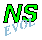## A dipole encounters a monopole

#### monopole nearly as strong / encounter with offset

A Lamb dipole with radius 0.75 and velocity of +2 (i.e. moving to the right) is placed to the left of a positive Bessel monopole with radius 0.50 at the origin.

In the main run of this example the dipole is along the x-axis, making the encounter head-on, and the monpole has a circulation of +4, which means that the monopole is weaker than each dipole half.

In this example:

• The circulation of the monopole is +8, which makes the monopole nearly as strong as each dipole half, since the circulation per dipole half is about 10.2.
• The dipole is located 0.5 above the x-axis: the encounter is with an offset.
The initial situation is shown in the following two graphs: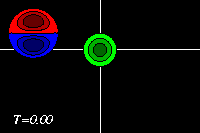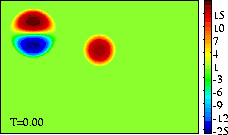Initial tracer (left) and vorticity (right) distribution.
The domain shown is x=[-3.0:+3.0], y=[-2.5:+1.5].
The extrema of voriticity are +/- 29.5 for the dipole
and +23.6 for the monopole.
The monopole has an angular momentum: it rotates about its central point, without moving. The dipole has a linear momentum: it moves in the direction of its axis, in this case to the right, towards the monopole.

The monopole rotates counter-clockwise, like the top half of the dipole. The result of the rotation is that the dipole is pushed down, to negative y-values, while it moves towards the monopole, like in the run with an offset and a weaker monopole. Since the monopole (green) is in this example stronger, the deformation of the positive dipole half (red) is larger. This can be seen clearest in the tracer plots: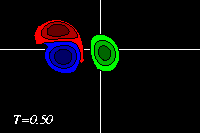Due to the approach of the dipole, the monopole is deformed slightly and pushed away from its initial location. The deformation of the positive dipole half (red) continues, while the monopole (green) comes close to the negative dipole half (blue).

The latter two form a dipolar structure, as in the run with an offset and a weaker monopole, but since the monopole is now nearly as strong as the dipole half, this newly formed dipolar structure moves away from, leaving the original positive dipole half (red) behind as a monopole (though the monopole is still "connected" through a thin vortex band with the dipole, as the red filled counter around the blue dipole half show):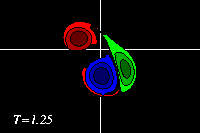The newly formed dipolar structure is not exactly symmetrical, so it moves along a curved path, with the stronger (negative) half on the inside: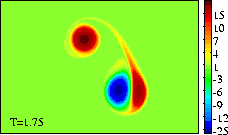These MPEG movies (81 frames; 456kb) show the evolution until T=2 more clearly:
===> MPEG movie of the tracer distribution
===> MPEG movie of the vorticity distribution

At initialisation, a single tracer particle was placed at the extrema of vorticity of both vortices. The next graph shows their trajectories:Trajectories of the tracers, initially placed at
the extrema of vorticity of the monopole (solid
line) and the dipole (dashed line).
Symbols are placed at intervals of T=0.25.

See the main run of this example, with a weaker monopole and a head-on encounter.

The evolution of the vorticity distribution is computed with a Finite Difference Method which solves the two-dimensional vorticity (Navier-Stokes) equation. Time and distances are given in dimensionless units.

===> Some details on the computation presented on this page for those who are interested.